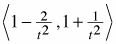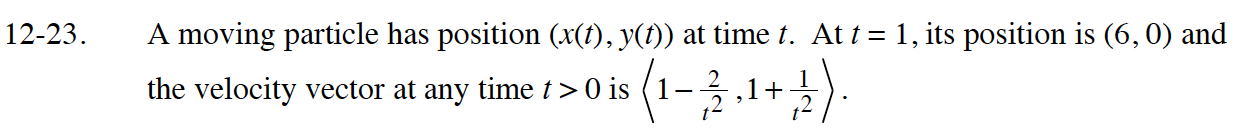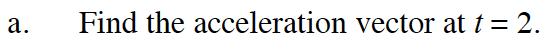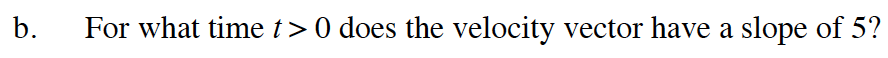### Home > CALC > Chapter 12 > Lesson 12.1.2 > Problem12-23

12-23.
1. A moving particle has position (x(t), y(t)) at time t. At t = 1, its position is (6, 0) and the velocity vector at any time t > 0 is. Homework Help ✎

1. Find the acceleration vector at t = 2.

2. For what time t > 0 does the velocity vector have a slope of 5?The acceleration vector can be obtained by taking the derivative of each component of the velocity vector.Divide the vertical component by the horizontal component of the velocity vector. This should equal 5.